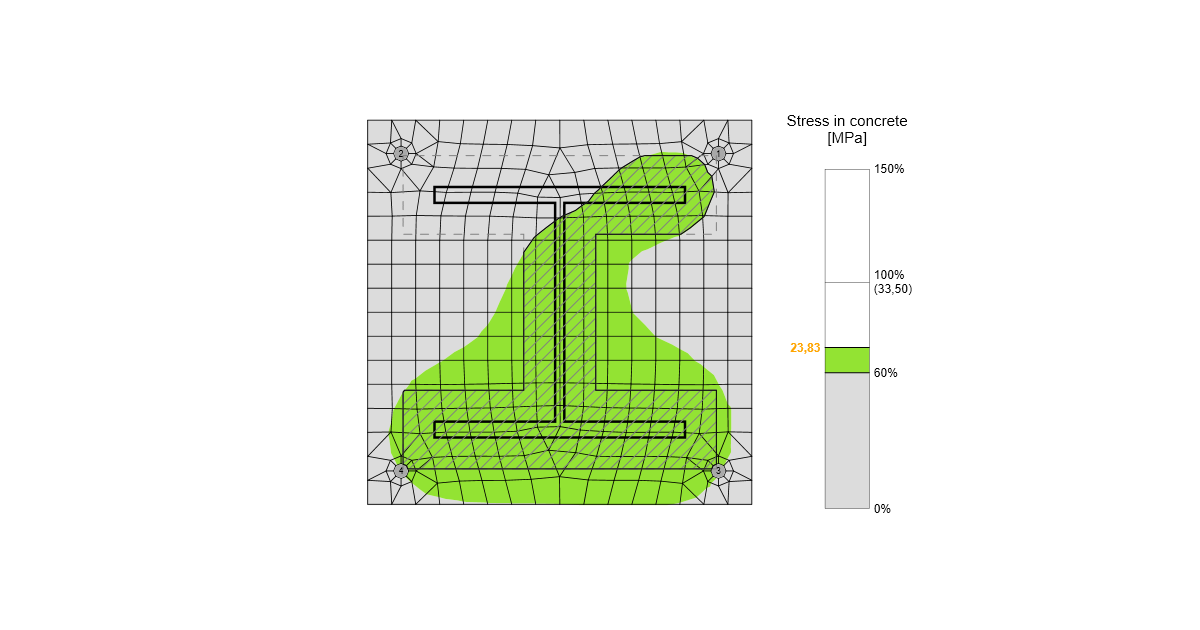### Choose language# Structural model of a concrete block

$$Design modelIn CBFEM, it is convenient to simplify the concrete block as 2D contact elements. The connection between the concrete and the base plate resists in compression only. Compression is transferred via the Winkler-Pasternak subsoil model, representing deformations of the concrete block. The tension force between the base plate and concrete block is carried by the anchor bolts. The shear force is transferred by friction between a base plate and a concrete block, by shear key and by bending of anchor bolts and friction. The resistance of bolts in shear is assessed analytically. Friction and shear key are modeled as a full single point constraint in the plane of the base plate – concrete contact.Deformation stiffnessThe stiffness of the concrete block may be predicted for the design of column bases as an elastic hemisphere. A Winkler-Pasternak subsoil model is commonly used for a simplified calculation of foundations. The stiffness of subsoil is determined using modulus of elasticity of concrete and the effective height of a subsoil as:$k = \frac{E_c}{(\alpha_1 + \upsilon) \sqrt{\frac{A_{eff}}{A_{ref}}}} \left( \frac{1}{\frac{h}{a_2 d} + a_3}+a_4 \right)$where: k – stiffness of concrete subsoil in compression Ec – modulus of elasticity of concrete υ – Poisson's coefficient of the concrete block Aeff – effective area in compression Aref = 1 m2 – reference area d – base plate width h – concrete block height a1 = 1.65; a2 = 0.5; a3 = 0.3; a4 = 1.0 – coefficients SI units must be used in the formula, the resulting unit is N/m3.Transfer of shear load at the base plateThe shear load at the base plate can be transferred by three means: Friction Shear lug Anchors Users can choose the mean by editing the base plate operation. No combination of means is allowed in the software, however, EN 1993-1-8 – Cl. 6.2.2 and Fib 58 – Chapter 4.2 allows for the combination of shear transfer by anchors and friction under certain conditions. In general, it is conservative to neglect friction in the anchorage design, although it may in some cases lead to an underestimation of concrete cracking at the serviceability level. As a rule, frictional resistance should be neglected if: the thickness of the grout layer exceeds one-half the anchor diameter, the anchorage capacity is governed by a near-edge condition, the anchorage is intended to resist earthquake loads. The combination with a shear lug should never be allowed due to the deformation compatibility.Transfer of shear load by frictionThe shear resistance equals the resistance safety factor multiplied by friction coefficient editable in Code setup and compressive load. The compressive load includes all forces, e.g. in case of a column base loaded by compressive force and bending moment, the compressive load used for frictional shear resistance might be higher than the applied compressive force.Transfer of shear load by shear lugThe shear lug is simulated as a stub encased in concrete under the base plate. The shear load is estimated to be transferred by uniform load distribution acting on the whole portion of the shear lug embedded in the concrete block, i.e. all nodes of the shear lug below the concrete surface are uniformly loaded. The portion of the shear lug above the concrete surface in grout is not assumed to transfer the shear load.Be aware that the lever arm between the applied shear load (at the base plate) and the shear resistance (half-height of the shear lug embedded in concrete) causes a bending moment which must be transferred by compressive force in concrete and tensile forces in anchors.The shear lug consists of shell finite elements and is checked as regular plates. Also, the welds of the shear lug to the base plate are checked using standard procedures in IDEA StatiCa Connection. Manual calculation usually assumes beam theory for the shear lug, although it is not accurate because the length to width ratio is very small for shear lug. Therefore, there might be a significant difference between IDEA StatiCa Connection and manual calculation.Transfer of shear load by anchorsThe shear resistance is determined by the shear resistance of anchors. The steel resistance of anchors have elastoplastic load-deformation curve, but the concrete failure modes are considered as perfectly brittle.$$